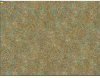# GMS 2Simple, pretty performant >TOP DOWN RAIN<

#### DukeSoft

##### Member
GM Version: Studio
Target Platform: Windows
Download: see code below
Links: N/A

Hey everyone,

I saw Cameron Penner 's top down rain video here;

And decided to add some improvements regarding speed (and added a wind variable).

Here goes;

obj_rain
create
Code:
``````rainlist = ds_list_create();
winddirection = random(360);
windspeed = 25; //Some arbitrary value. 100 is a lot of wind. 0 is none.
length = 4;
edge = 50; //How far outside of the view should we create drops
max_raindrops = 1000;
show_debug_overlay(1);
var xx = camera_get_view_x(view_camera);
var yy = camera_get_view_y(view_camera);
var w = camera_get_view_width(view_camera);
var h = camera_get_view_height(view_camera);

//Fill up the list initally
while (ds_list_size(rainlist)/3 < max_raindrops) {
ds_list_add(rainlist, xx-edge+random(w+edge*2)); //X
ds_list_add(rainlist, yy-edge+random(h+edge*2)); //Y
ds_list_add(rainlist, random(50));  //Random starting values
}``````
draw
Code:
``````var xx = camera_get_view_x(view_camera);
var yy = camera_get_view_y(view_camera);
var w = camera_get_view_width(view_camera);
var h = camera_get_view_height(view_camera);

var px = lengthdir_x(windspeed/10, winddirection);
var py = lengthdir_y(windspeed/10, winddirection);

while (ds_list_size(rainlist)/3 < max_raindrops) { //Fill the list if it got empty
ds_list_add(rainlist, xx-edge+random(w+edge*2)); //X
ds_list_add(rainlist, yy-edge+random(h+edge*2)); //Y
ds_list_add(rainlist, 40+random(10));  //Z
}

var vx, vy;
var halfw = w/2+lengthdir_x(windspeed*2, winddirection);
var halfh = h/2+lengthdir_y(windspeed*2, winddirection);
draw_set_color(c_aqua);
draw_set_alpha(0.6);

for (var i = 0; i < (ds_list_size(rainlist)/3); i++;) {
var ii = (i*3);
rainlist[| ii+2] -= 1; //Z
rainlist[| ii] += px;
rainlist[| ii+1] += py;

var xxx = rainlist[| ii];
var yyy = rainlist[| ii+1];
var zzz = rainlist[| ii+2];

if (zzz < 0) {
instance_create_depth(xxx, yyy, 10, obj_raindrop); ////// <- you're gonna need a raindrop object if you want it
ds_list_delete(rainlist, ii);
ds_list_delete(rainlist, ii+1);
ds_list_delete(rainlist, ii+2);
} else {
vx = (xxx - (xx+halfw))/halfw;
vy = (yyy - (yy+halfh))/halfh;
var sqz1 = sqr(zzz);
var sqz2 = sqr(zzz+length);
draw_line_width(
xxx + vx*sqz1,
yyy + vy*sqz1,
xxx + vx*sqz2,
yyy + vy*sqz2,
2
);
}
}``````
Example:Now bear in mind that this is probably better to do with a shader, but for a small and funny effect (and much less cost than the solution from the video) you've got some top-down rain.

Feel free to improve, share, edit, whatever you likeLast edited by a moderator:
••Alicja, SilentxxBunny and (deleted member)